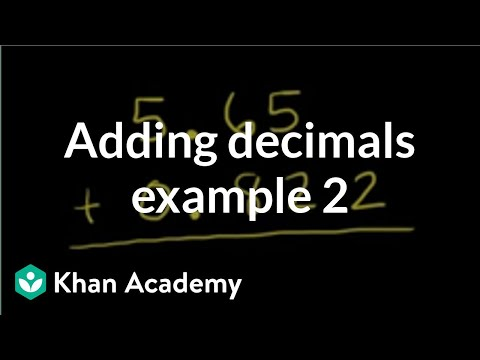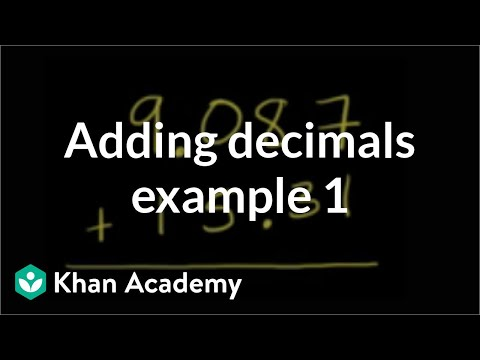Video

# Dividing a whole number by a decimal (Full video)

Khan Academy

## Supporting grades: 6

Description: When dividing a whole number by a decimal, you're going to need to change that decimal into a whole number. How? We'll show you. Created by Sal Khan. So we're dividing this whole number by a decimal. Let me write a little bit bigger than that, since we have to do some work with it. So the first thing we do, since we have this decimal here, we're dividing by a decimal.

You must log inorsign upif you want to:*

*Teacher Advisor is 100% free.

### Other videos you might be interested in### Adding decimals: 0.822 + 5.65 (Full video)

#### Khan Academy